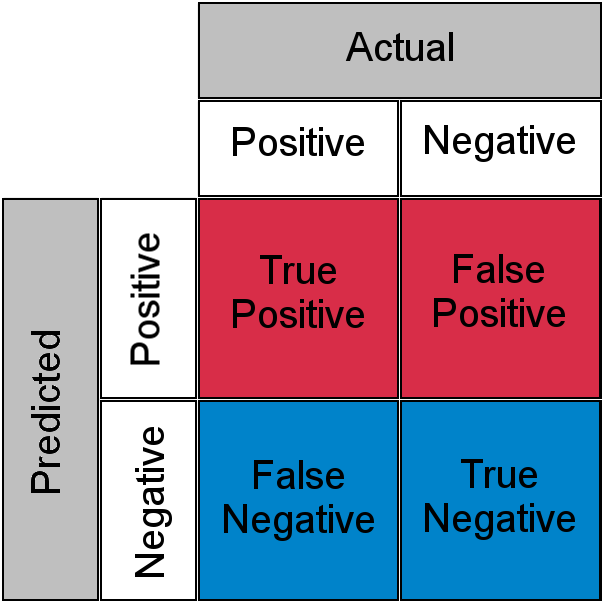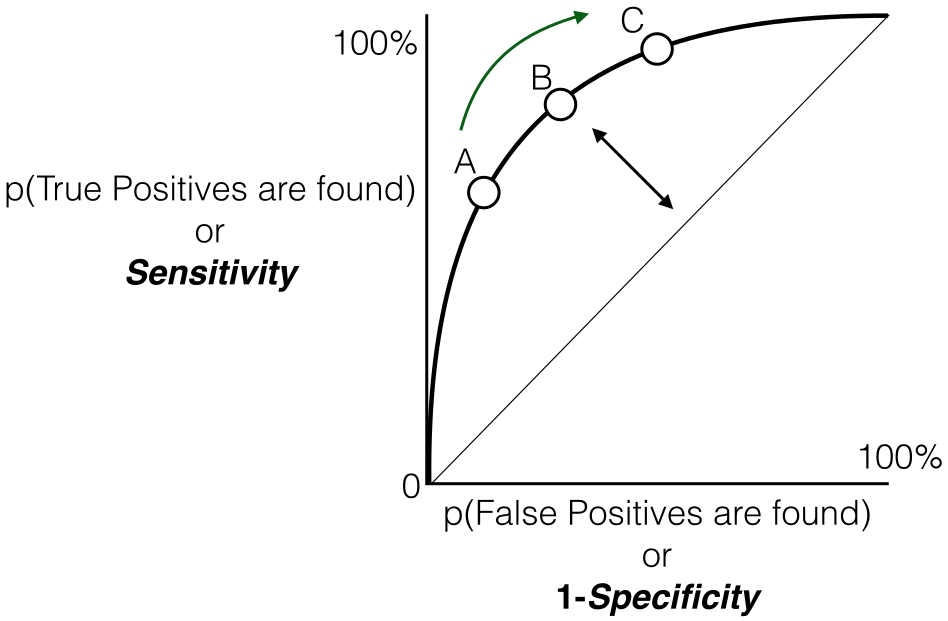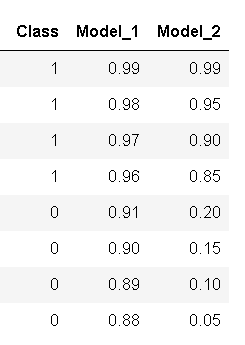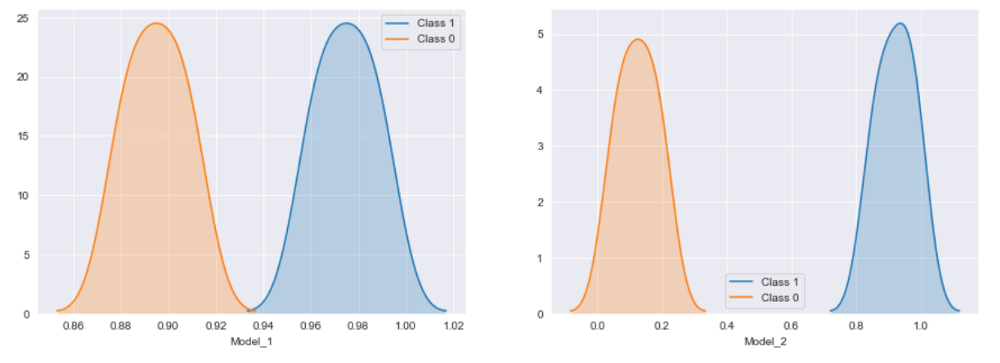Skip to content
Related Articles
AUC-ROC Curve
• Difficulty Level : Medium
• Last Updated : 26 Nov, 2020

One important aspect of Machine Learning is model evaluation. You need to have some mechanism to evaluate your model. This is where these performance metrics come into the picture they give us a sense of how good a model is. If you are familiar with some basics of Machine Learning then you must have across some of these metrics like accuracy, precision, recall, auc-roc, etc.

Let’s say you are working on a binary classification problem and come up with a model with 95% accuracy, now someone asks you what does that mean you would be quick enough to say out of 100 predictions your model makes, 95 of them are correct. Well lets notch it up a bit, now the underlying metric is recall and you are asked the same question, you might take a moment here but eventually, you would come up with an explanation like out of 100 relevant data points(positive class in general) your model is able to identify 80 of them. So far so good, now let us assume you evaluated your model using AUC-ROC as a metric and got a value of 0.75 and again I shoot the same question at you what does 0.75 or 75% signify, now you might need to give it a thought, some of you might say there is a 75% chance that model identifies a data point correctly but by now you would have already realized that’s not it. Let us try to get a basic understanding of one the most used performance metrics out there for classification problems.

History:

If you have participated in any online machine learning competition/hackathon then you must have come across Area Under Curve Receiver Operator Characteristic a.k.a AUC-ROC, many of them have it as their evaluation criteria for their classification problems. Let’s admit when you had first heard about it, this thought once must have crossed your mind, what’s with the long name? Well, the origin of ROC curve goes way back in World War II, it was originally used for the analysis of radar signals. The United States Army tried to measure the ability of their radar receiver to correctly identify the Japanese Aircraft. Now that we have a bit of origin story lets get down to business

Geometric Interpretation:

This is the most common definition that you would have encountered when you would Google AUC-ROC. Basically, ROC curve is a graph that shows the performance of a classification model at all possible thresholds( threshold is a particular value beyond which you say a point belongs to a particular class). The curve is plotted between two parameters

• TRUE POSITIVE RATE
• FALSE POSTIVIE RATE

Before understanding, TPR and FPR let us quickly look at the confusion matrix.Source: Creative Commons

• True Positive: Actual Positive and Predicted as Positive
• True Negative: Actual Negative and Predicted as Negative
• False Positive(Type I Error): Actual Negative but predicted as Positive
• False Negative(Type II Error): Actual Positive but predicted as Negative

In simple terms, you can call False Positive as false alarm and False Negative as a miss. Now let us look at what TPR and FPR.Basically TPR/Recall/Sensitivity is ratio of positive examples that are correctly identified and FPR is the ratio of negative examples that are incorrectly classified.

and as said earlier ROC is nothing but the plot between TPR and FPR across all possible thresholds and AUC is the entire area beneath this ROC curve.Source: Creative Commons

PROBABILISITC INTERPRETION:

We looked at the geometric interpretation, but I guess it is still not enough in developing the intuition behind what does 0.75 AUC actually means, now let us look at AUC-ROC with a probabilistic point of view.

Let me first talk about what AUC does and later we will build our understanding on top of this

AUC measures how well a model is able to distinguish between classes

An AUC of 0.75 would actually mean that let’s say we take two data points belonging to separate classes then there is 75% chance model would be able to segregate them or rank order them correctly i.e positive point has a higher prediction probability than the negative class. (assuming a higher prediction probability means the point would ideally belong to the positive class)

Here is a small example to make things more clear.

Here we have 6 points where P1, P2, P5 belong to class 1 and P3, P4, P6 belong to class 0 and we’re corresponding predicted probabilities in the Probability column, as we said if we take two points belonging to separate classes then what is the probability that model rank orders them correctly

We will take all possible pairs such that one point belongs to class 1 and other belongs to class 0, we will have total 9 such pairs below are all of these 9 possible pairs

Here column isCorrect tells if the mentioned pair is correct rank-ordered based on the predicted probability i.e class 1 point has a higher probability than class 0 point, in 7 out of these 9 possible pairs the class 1 is ranked higher than class 0, or we can say that there is a 77% chance that if you pick a pair of points belonging to separate classes the model would be able to distinguish them correctly. Now, I think you might have a bit intuition behind this AUC number, just to clear up any further doubts lets validate it using scikit learn’s AUC-ROC implementation

Python implementation code:

 `import` `numpy as np `` ` `from` `sklearn .metrics ``import` `roc_auc_score `` ` `y_true ``=` `[``1``, ``1``, ``0``, ``0``, ``1``, ``0``]`` ` `y_pred ``=` `[``0.95``, ``0.90``, ``0.85``, ``0.81``, ``0.78``, ``0.70``]`` ` `auc ``=` `np.``round``(roc_auc_score(y_true, y_pred), ``3``) `` ` `print``(``"Auc for our sample data is {}"``. ``format``(auc))`

When to use:

Having said that there certain places where ROC-AUC might not be ideal.

• ROC-AUC does not work well under severe imbalance in the dataset, to give some intuition for this lets us look back at the geometric interpretation here. Basically, ROC is the plot between TPR and FPR( assuming the minority class is a positive class), now let us have a close look at the FPR formula againDenominator of FPR has a True Negatives as one factor since Negative Class is in majority the denominator of FPR is dominated by True Negatives which makes FPR less sensitive to any changes in minority class predictions. To overcome this, Precision-Recall Curves are used instead of ROC and then the AUC is calculated, try to answer this yourself how does Precision-Recall curve handle this problem (Hint: Recall and TPR are same technically only FPR is replaced with Precision, just compare the denominators for both and try to assess how imbalance problem is solved here)

• ROC-AUC tries to measure if the rank ordering of classifications is correct it does not take into account actually predicted probabilities, let me try to make this point clear with a small code snippet
 `import` `pandas as pd`` ` `y_pred_1 ``=` `[``0.99``, ``0.98``, ``0.97``, ``0.96``, ``0.91``, ``0.90``, ``0.89``, ``0.88``]``y_pred_2 ``=` `[``0.99``, ``0.95``, ``0.90``, ``0.85``, ``0.20``, ``0.15``, ``0.10``, ``0.05``]``y_act ``=` `[``1``, ``1``, ``1``, ``1``, ``0``, ``0``, ``0``, ``0``]``test_df ``=` `pd.DataFrame(``zip``(y_act, y_pred_1, y_pred_2),``                       ``columns``=``[``'Class'``, ``'Model_1'``, ``'Model_2'``])`Class Probabilities for two sample models

We have two models Model_1 and Model_2 as mentioned above, both do a perfect job in segregating the two classes, but if I ask you to choose one among them which one would it be, hold on to your answer let me first plot these model probabilities.

 `import` `matplotlib.pyplot as plt`` ` `cols ``=` `[``'Model_1'``, ``'Model_2'``]``fig, axs ``=` `plt.subplots(``1``, ``2``, figsize``=``(``15``, ``5``))``for` `index, col ``in` `enumerate``(cols):``    ``sns.kdeplot(d2[d2[``'Status'``] ``=``=` `1``][col],``                ``label``=``"Class 1"``, shade``=``True``, ax``=``axs[index])``    ``sns.kdeplot(d2[d2[``'Status'``] ``=``=` `0``][col],``                ``label``=``"Class 0"``, shade``=``True``, ax``=``axs[index])``    ``axs[index].set_xlabel(col)``plt.show()`Class Probability Distribution for sample models

If there were any slightest of doubts earlier, I guess now your choice would quite clear, Model_2 is a clear winner. But the AUC-ROC values would be same for both, this is the drawback it just measures if the model is able to rank order the classes correctly it does not look at how well the model separates the two classes, hence if you have a requirement where you want to use the actually predicted probabilities then roc might not be the right choice, for those who are curious log loss is one such metric that solves this problem

So ideally one should use AUC when there dataset does not have a severe imbalance and when your use case does not require you to use actual predicted probabilities.

AUC FOR MULTI-CLASS:

For a multi-class setting, we can simply use one vs all methodology and you will have one ROC curve for each class. Let’s say you have four classes A, B, C, D then there would ROC curves and corresponding AUC values for all the four classes, i.e. once A would be one class and B, C and D combined would be the others class, similarly B is one class and A, C and D combined as others class, etc.My Personal Notes arrow_drop_up